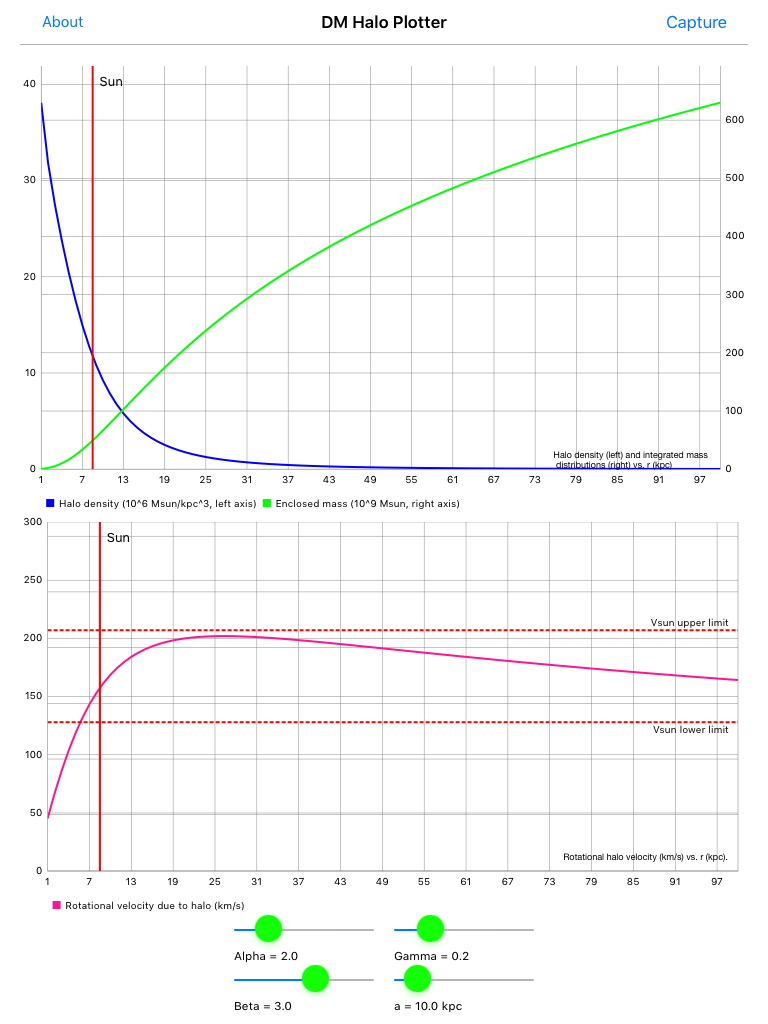# DMHaloPlotterThis App was developed to visualize the dark matter halo in the Milky Way galaxy and use that distribution to determine the rotational velocity of objects in our galaxy due to the halo.

The dark matter halo distribution in the Milky Way galaxy has been described by Lars Bergstrom, Piero Ullio, and James H. Buckley in http://arxiv.org/abs/astro-ph/9712318. The halo distribution is characterized by 4 parameters: alpha, beta, gamma, and the core radius a. These parameters can be changed using the sliders. The default parameters are alpha = 2, beta = 3, gamma = 0.2, and a = 10 kpc.

The halo distribution is constrained by the boundary condition that the total mass of the dark matter halo with 100 kpc is (6.3 +/- 2.5)x10^11 solar masses. The halo density and the intergrated mass distributions are normalized to satisfy this boundary condition.

The shape of the halo distribution is further constrained by the measured rotational velocity of the sun. The following constraints are shown in the graph of the rotational velocity:

vh(R0) = 128 − 207 km/s if R0 = 8.5 kpc

vh(R0) = 86 - 149 km/s if R0 = 7.1 kpc

where vh is the rotational velocity of the sun due to the dark matter halo and R0 is radial position of the sun.

Link to the App on the AppStore

Developers: Jun Yin and Frank Wolfs

Contact Information: yjwhlf@gmail.com